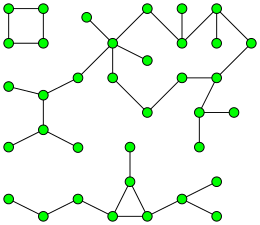# Connected component (graph theory)A graph with three connected components.

In graph theory, a connected component (or just component) of an undirected graph is a subgraph in which any two vertices are connected to each other by paths, and which is connected to no additional vertices in the supergraph. For example, the graph shown in the illustration on the right has three connected components. A vertex with no incident edges is itself a connected component. A graph that is itself connected has exactly one connected component, consisting of the whole graph.

## An equivalence relation

An alternative way to define connected components involves the equivalence classes of an equivalence relation that is defined on the vertices of the graph. In an undirected graph, a vertex v is reachable from a vertex u if there is a path from u to v. In this definition, a single vertex is counted as a path of length zero, and the same vertex may occur more than once within a path. Reachability is an equivalence relation, since:

• It is reflexive: There is a trivial path of length zero from any vertex to itself.
• It is symmetric: If there is a path from u to v, the same edges form a path from v to u.
• It is transitive: If there is a path from u to v and a path from v to w, the two paths may be concatenated together to form a path from u to w.

The connected components are then the induced subgraphs formed by the equivalence classes of this relation.

## The number of connected components

The number of connected components is an important topological invariant of a graph. In topological graph theory it can be interpreted as the zeroth Betti number of the graph. In algebraic graph theory it equals the multiplicity of 0 as an eigenvalue of the Laplacian matrix of the graph. It is also the index of the first nonzero coefficient of the chromatic polynomial of a graph. Numbers of connected components play a key role in the Tutte theorem characterizing graphs that have perfect matchings, and in the definition of graph toughness.

## Algorithms

It is straightforward to compute the connected components of a graph in linear time (in terms of the numbers of the vertices and edges of the graph) using either breadth-first search or depth-first search. In either case, a search that begins at some particular vertex v will find the entire connected component containing v (and no more) before returning. To find all the connected components of a graph, loop through its vertices, starting a new breadth first or depth first search whenever the loop reaches a vertex that has not already been included in a previously found connected component. Hopcroft and Tarjan (1973) describe essentially this algorithm, and state that at that point it was "well known".

There are also efficient algorithms to dynamically track the connected components of a graph as vertices and edges are added, as a straightforward application of disjoint-set data structures. These algorithms require amortized O(α(n)) time per operation, where adding vertices and edges and determining the connected component in which a vertex falls are both operations, and α(n) is a very slow-growing inverse of the very quickly growing Ackermann function. A related problem is tracking connected components as all edges are deleted from a graph, one by one; an algorithm exists to solve this with constant time per query, and O(|V||E|) time to maintain the data structure; this is an amortized cost of O(|V|) per edge deletion. For forests, the cost can be reduced to O(q + |V| log |V|), or O(log |V|) amortized cost per edge deletion.

Researchers have also studied algorithms for finding connected components in more limited models of computation, such as programs in which the working memory is limited to a logarithmic number of bits (defined by the complexity class L). Lewis & Papadimitriou (1982) asked whether it is possible to test in logspace whether two vertices belong to the same connected component of an undirected graph, and defined a complexity class SL of problems logspace-equivalent to connectivity. Finally Reingold (2008) succeeded in finding an algorithm for solving this connectivity problem in logarithmic space, showing that L = SL.